Question

# 9. Find the amount of current in the resistor of problem 8 at t = 2.50s....

9. Find the amount of current in the resistor of problem 8 at t = 2.50s. 10. Three 0.01Ω resistors which are connected in parallel are powered by a battery with E = 10.0V. What is the internal resistance of the battery if I = 3.03 ⋅ 10−2 A in this circuit?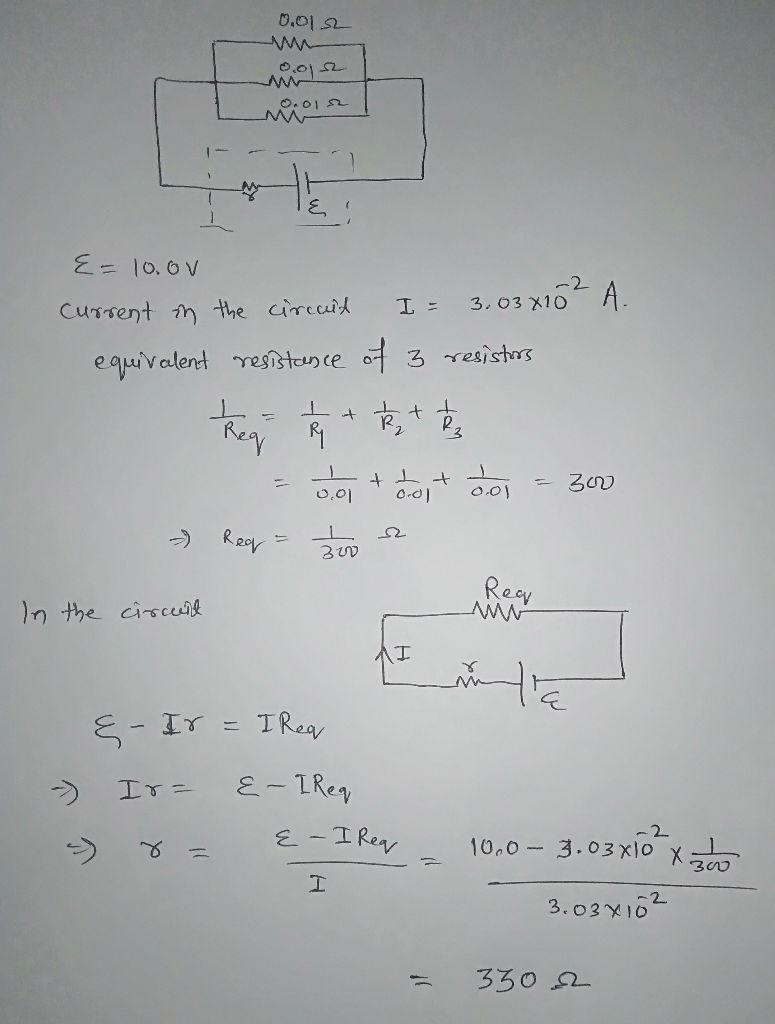#### Earn Coins

Coins can be redeemed for fabulous gifts.

Similar Homework Help Questions
• ### A 1.40 kΩ resistor is connected in series with a 0.800 kΩ resistor. This pair of...

A 1.40 kΩ resistor is connected in series with a 0.800 kΩ resistor. This pair of resistors is connected in parallel with a 7.00 kΩ resistor. That combination is then connected in series with a 0.700 kΩ resistor and a 9.0-V ideal battery (no internal resistance of course). A) sketch this circuit B) Determine the total equivalent resistance of this circuit's arrangement C) Determine the amount of current that flows through the battery D) Determine the voltage drop across the...

• ### Three 4.3 Ω resistors are connected in series with a 16.0 V battery. Find the following....

Three 4.3 Ω resistors are connected in series with a 16.0 V battery. Find the following. (a) the equivalent resistance of the circuit (b) the current in each resistor (c) Repeat for the case in which all three resistors are connected in parallel across the battery. equivalent resistance ______ current in each resistor _______

• ### Three 4.3 Ω resistors are connected in series with a 16.0 V battery. Find the following....

Three 4.3 Ω resistors are connected in series with a 16.0 V battery. Find the following. (a) the equivalent resistance of the circuit (b) the current in each resistor (c) Repeat for the case in which all three resistors are connected in parallel across the battery. equivalent resistance ______ current in each resistor _______

• ### PHYSICS II: 4) For the circuit shown below, find: a) the equivalent resistance; b) the current through the battery;...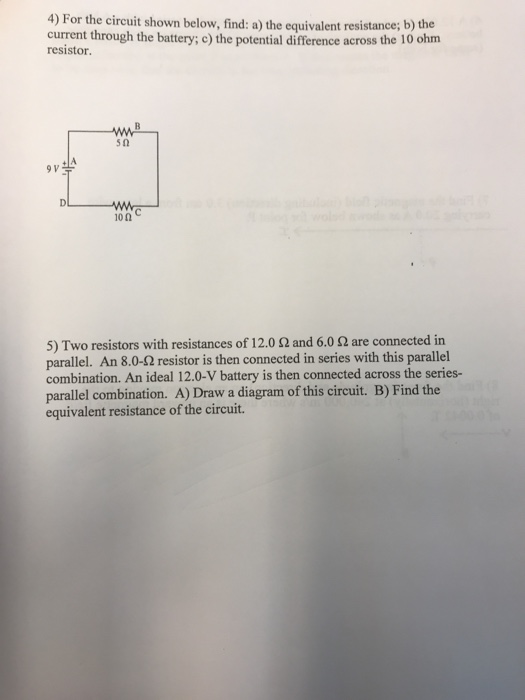PHYSICS II: 4) For the circuit shown below, find: a) the equivalent resistance; b) the current through the battery; c) the potential difference across the 10 ohm resistor. 9 p /A we 100C 5) Two resistors with resistances of 12.0 2 and 6.0 are connected in parallel. An 8.0-12 resistor is then connected in series with this parallel combination. An ideal 12.0-V battery is then connected across the series- parallel combination. A) Draw a diagram of this circuit. B) Find...

• ### A potentiometer is essentially a resistor with three contacts, one of which is mobile. It acts...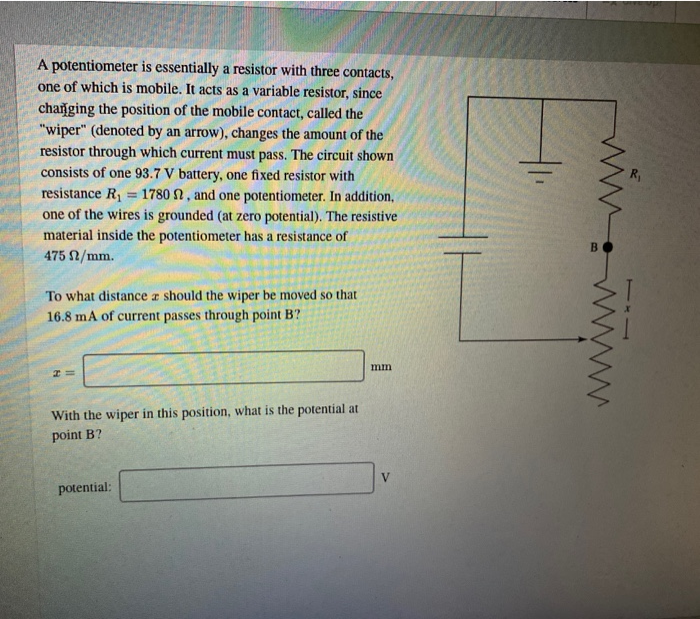A potentiometer is essentially a resistor with three contacts, one of which is mobile. It acts as a variable resistor, since changing the position of the mobile contact, called the "wiper" (denoted by an arrow), changes the amount of the resistor through which current must pass. The circuit shown consists of one 93.7 V battery, one fixed resistor with resistance Ri = 1780 N, and one potentiometer. In addition, one of the wires is grounded (at zero potential). The resistive...

• ### What is the current through the 8 resistor in the cicrcuit in problem 11 of a...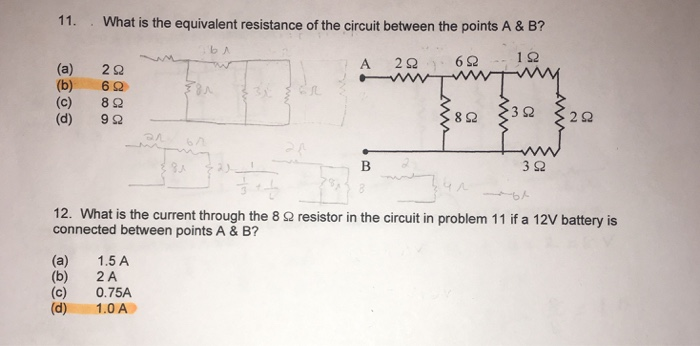What is the current through the 8 resistor in the cicrcuit in problem 11 of a 12V battery is connected between points A & B? • based off of my answer on #11 11. What is the equivalent resistance of the circuit between the points A & B? (a) (b) (c) (d) 2Ω 6Ω 8Ω 9Ω 3Ω 12. What is the current through the 8 Ω resistor in the circuit in problem 11 if a 12V battery is connected between...

• ### Learning Goal: To practice Problem-Solving Strategy 23.1 Resistor Circuits. Find the currents through and the potential...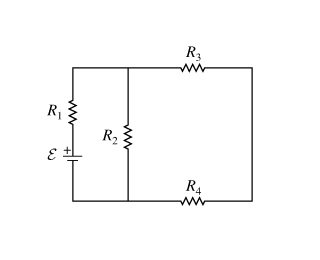Learning Goal: To practice Problem-Solving Strategy 23.1 Resistor Circuits. Find the currents through and the potential difference across each resistor in the circuit shown on the diagram (Figure 1) . Use the following values: E = 12.0V , R1 = 15.0? , R2 = 45.0? , R3 = 20.0? , and R4 = 25.0? . PROBLEM-SOLVING STRATEGY 23.1 Resistor Circuits We can analyze any resistor circuit by sequentially reducing parallel and series resistor combinations to their equivalent resistors until only...

• ### Part C: Post Lab Questions for Lab 9 Complete the Lab before you work on these...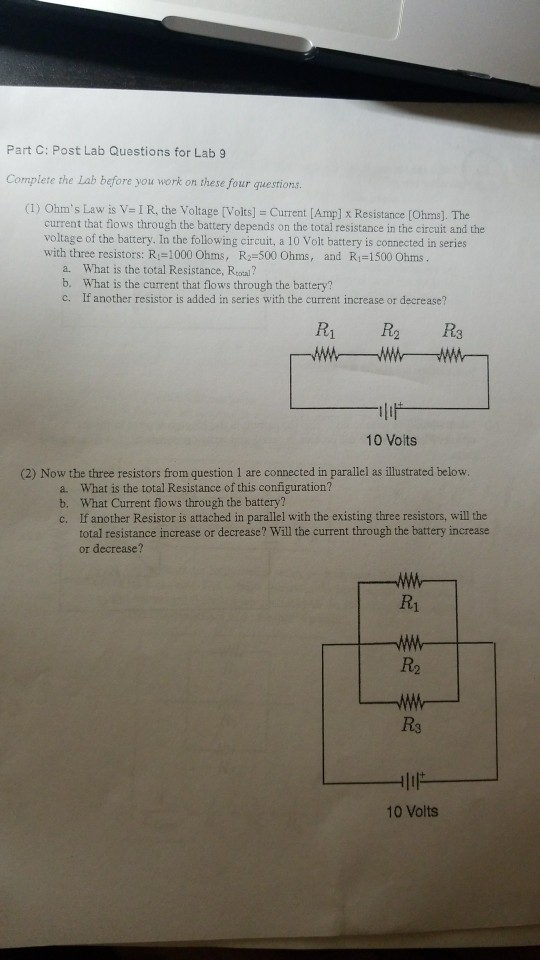Part C: Post Lab Questions for Lab 9 Complete the Lab before you work on these four questions (1) Ohm's Law is v= 1 R, the Voltage [Volts] = Current [Amp] x Resistance [Ohms]. The current that flows through the battery depends on the total resistance in the circuit and the voltage of the battery. In the following circuit, a 10 Volt battery is connected in series with three resistors: Ri-1000 Ohms, R-500 Ohms, and R1-1500 Ohms a. What is...

• ### 1. Find the capacitance of a conductive sphere of radius 1.0fm charged with 1.60. 10-19C. 2. Now ...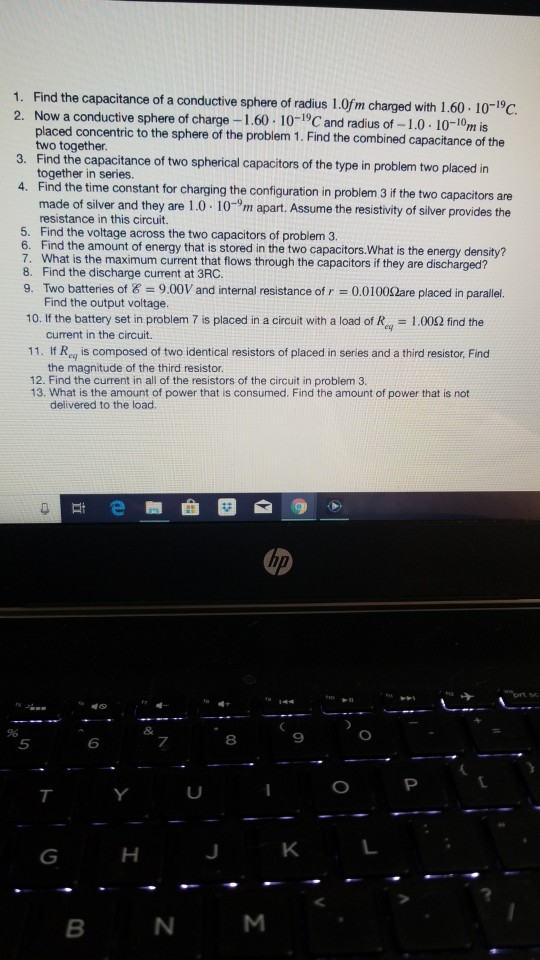number 3 1. Find the capacitance of a conductive sphere of radius 1.0fm charged with 1.60. 10-19C. 2. Now a conductive sphere of charge -I placed concentric to the sphere of the problem 1. Find the combined capacitance of the two together. 3. Find the capacitance of two spherical capacitors of the type in problem two placed in together in series. 4. Find the time constant for chargingthe configuratonin problem Зі the two capacitors are made of siver and they...

• ### A battery having an emf of 9.03 V delivers 120 mA when connected to a 62.5...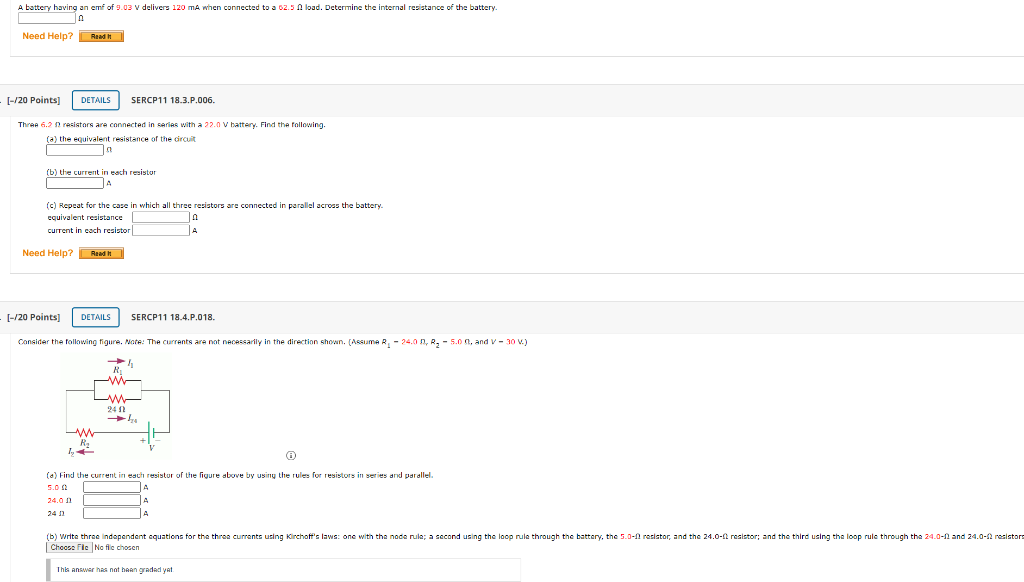A battery having an emf of 9.03 V delivers 120 mA when connected to a 62.5 load. Determine the internal resistance of e battery Need Help? Read [-720 Points] DETAILS SERCP11 18.3.P.006. Three 6.2 resistors are connected in series with a 2.0 V battery. Find the following. (a) the equivalent resistance of the circuit (b) the current in each resistor (c) Repeat for the case in which all three resistors are connected in parallel across the battery. equivalent resistance current...